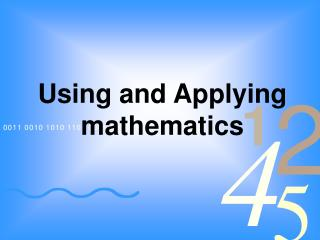Download PresentationUsing and Applying mathematicsUsing and Applying mathematics - PowerPoint PPT Presentation

Download PresentationUsing and Applying mathematics
An Image/Link below is provided (as is) to download presentation

Download Policy: Content on the Website is provided to you AS IS for your information and personal use and may not be sold / licensed / shared on other websites without getting consent from its author. While downloading, if for some reason you are not able to download a presentation, the publisher may have deleted the file from their server.

- - - - - - - - - - - - - - - - - - - - - - - - - - - E N D - - - - - - - - - - - - - - - - - - - - - - - - - - -
Presentation Transcript

1. Using and Applying mathematics

2. Aims: • To consider the different elements of the Using and Applying strand • To understand the progression within each of the elements • To know the 5 different types of problem • To understand the range of strategies needed to solve problems

3. Quick and easy Using and Applying examples. • Jemma thinks of a number. She says, 'Add 3 to my number and then multiply the result by 5. The answer is 35'. What is Jemma's number? • I multiply two numbers and the answer is 48 • Odd one out: 1 4 19 25 • Odd one out:square, rectangle, equilateral triangle, trapezium • Can you join all 9 dots using only four straight lines (you must not take your pencil off the page)? • • • • • • • • •

4. Using and applying mathematics in the electronic Framework • One of the aims of the Primary Framework is to give greater attention to using and applying mathematics. The Using and applying mathematics strand has five themes and there is progression built into each theme. • The five themes are: • Solving problems • Representing – analyse, record, do, check, confirm • Enquiring – plan, decide, organise, interpret, reason, justify • Reasoning – create, deduce, apply, explore, predict, hypothesise, • test • Communicating – explain methods and solutions, choices, • decisions, reasoning

5. Solving problems

6. Possible barriers for children when problem solving could be: • Unfamiliarity with this type of problem; • Relying on trial and error and hit-and-miss strategies; • Thinking that there was only one solution; • Not reading the problem carefully enough; • Not recording carefully enough the data they generated; • Not talking about the problem with other children; • Not annotating the problem sheet; • Not being secure enough with the strategies.

7. Types of problems. • Word problems • Finding all possibilities • Logic puzzles • Finding rules and describing patterns • Diagram problems and visual puzzles

8. Word Problems • Choose and do a calculation • Use the result of the calculation to answer the problem

9. Finding all possibilities • Systematic – generating lists and organising/recording data • Need to sort the information • No calculations identified in the problem • More than one answer

10. Logic puzzles • No calculations involved • Given just enough information to solve • The challenge is to hold one piece of information while interpreting another

11. Finding rules and describing patterns • Develops reasoning skills • Children may develop strategies as they progress • Children may not find the most efficient way

12. Diagram problems and visual puzzles • Important to be systematic • Develops a range of ways of recording

13. Do you provide equal teaching and learning opportunities for each of the five elements of the Using and Applying strand and for each of the five different types of word problems?